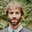Question-and-Answer Resource for the Building Energy Modeling Community
Get started with the Help page

# Energyplus FMU issuesHi everyone,

I am trying to run a FMU from E+. I got this problem in the log file and I do not have a clue what it is happening. I am running this in a container using Docker (EnergyPlus 9.3, .IDF Version 9.3) and PyFMI.

FMIL: module = FMI2XML, log level = 3: fmi2_xml_get_default_experiment_start: returning default value, since no attribute was defined in modelDescription

The model is correctly initialized

lab = load_fmu("Lab_IRH.fmu",log_level=4)

days=60

final_time = days*24*60*60

start_time = 0

lab.setup_experiment(stop_time=final_time)


### But when I try with both of the below setups

Turns out this one give me only zeros at the output of the FMU

stepsize=int(10*60)

i=0

while i<final_time:

status=lab.do_step(current_t=i,step_size=stepsize,new_step=True)

print(lab.get("TRooMea"))

i=i+stepsize


And this one got stuck infinitely bringing the error above mentioned

test=np.ones(96)*1000

u_traj = np.vstack(test).transpose()

inputs=['Q']

input_object = (inputs, u_traj)

opts=lab.simulate_options()

opts["ncp"] = 600

lab.simulate(final_time=final_time, options=opts)


The socket file is correctly generated and there is not information in the .err generated file. It must be probably something in the modeDescription.xml file in the FMU, but I am not sure. Do you know what can be causing the problem?

edit retag close merge delete

Sort by » oldest newest most voted

I think it may be because of a missing function call. I don't see your FMU been initialized.

I suggest for the first setup to replace the lab.setup_experiment(stop_time=final_time) with lab.initialize(start_time=start_time, stop_time=final_time).

For the second setup, just get rid of the lab.setup_experiment(stop_time=final_time) and modify your call to the simulate function to be lab.simulate(start_time=start_time, final_time=final_time, options=opts).

I am assuming that have used the latest version of EnergyPlusToFMU to export your FMU and that the IDF step size is 10.

more